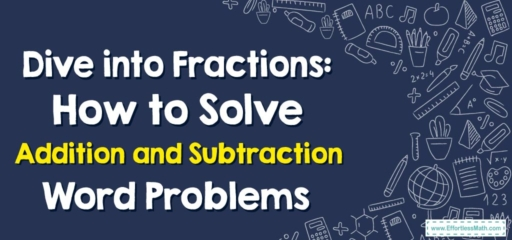# Dive into Fractions: How to Solve Addition and Subtraction Word Problems

Welcome back, budding mathematicians! Today, we'll explore a new exciting topic: word problems involving addition and subtraction of fractions. Don't let the term 'word problems' intimidate you. With some simple steps, you can tackle these like a pro.## Adding and Subtracting Fractions: A Refresher

Adding and subtracting fractions involves a little more than adding and subtracting whole numbers, but the concept is pretty straightforward. It revolves around the idea of finding a common denominator, which is a shared multiple of the denominators of the fractions involved.

## Step-by-Step Guide: Adding and Subtracting Fractions in Word Problems

### Step 1: Understand the Problem

Read the problem carefully. Understand what is being asked and identify the fractions you will need to add or subtract.

### Step 2: Find the Common Denominator

This is perhaps the trickiest part. The denominators of the fractions must be the same before you can add or subtract them. To find the common denominator, look for the least common multiple (LCM) of the denominators.

### Step 3: Adjust the Fractions

Once you have the common denominator, adjust the fractions by multiplying the numerator and denominator by the same number so that the denominator matches the common denominator.

### Step 4: Add or Subtract

Now, add or subtract the numerators of the fractions. The denominator remains the same.

### Step 5: Simplify the Answer

After performing the operation, simplify your answer if possible. This may involve reducing the fraction to its lowest term.

### Step 6: Write the Answer

Now, let’s see this process in action:

Ella has $$\frac{2}{3}$$ of a chocolate bar left, and her friend Mia has $$\frac{1}{2}$$ of a chocolate bar left. How much chocolate do they have together?

1. Understand the problem: We need to add $$\frac{2}{3}$$ and $$\frac{1}{2}$$.
2. Find the common denominator: The LCM of $$3$$ and $$2$$ is $$6$$.
3. Adjust the fractions: $$\frac{2}{3}$$ becomes $$\frac{4}{6}$$, and $$\frac{1}{2}$$ becomes $$\frac{3}{6}$$.
4. Add the fractions: $$\frac{4}{6} + \frac{3}{6} = \frac{7}{6}$$.
5. Simplify the answer: $$\frac{7}{6}$$ can be written as $$1\frac{1}{6}$$.
6. Write the answer: Ella and Mia have $$1\frac{1}{6}$$ chocolate bars together.

Remember, the key to mastering word problems involving fractions is practice. So, roll up your sleeves and dive in!

### What people say about "Dive into Fractions: How to Solve Addition and Subtraction Word Problems - Effortless Math: We Help Students Learn to LOVE Mathematics"?

No one replied yet.

X
30% OFF

Limited time only!

Save Over 30%

SAVE $5 It was$16.99 now it is \$11.99